Next: References Up: Longitudinal electrodynamic forces Previous: Summary

# Notation

The following notation is used throughout the report:

• Vectors are denoted by boldface fonts, as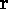and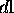. Unit vectors have a hat on top of them, as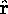.
• Scalar quantities are written with normal italic fonts, as I and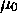. The same goes for the absolute length of a vector, i.e.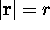• r (which appears e.g. in the discussion on Maxwell stresses) means the radial distance from the center, in cylindrical coordinates.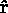is the unit vector in the radial direction.
• Not to be confused with the above,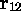is the vector from point (current element) 1 to point (current element) 2;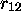is the length of this vector; and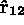is the unit vector in its direction.
•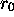is the distance from the origin. (It is used in the discussion on surface charges.)

# A derivation of Grassmann's law

Biot-Savart's law, well known from magnetostatics, gives the magnetic field from a circuit: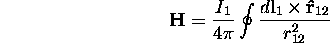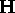is the magnetic field;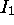the electric current;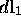is an infinitesimal section of the conductor;the distance fromto the point where the magnetic field is to be measured,; andthe unit vector fromto that point.

In differential form it becomes: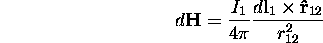The magnetic force (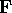) that moving charge experiences in a magnetic field is given by the Lorentz force law: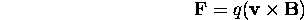Here q is the charge that is moving;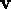its velocity; and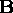the magnetic induction. With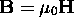in vacuum (and air), and noting that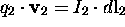we have: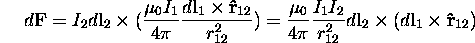which is Grassmann's law for the force between two current elements.

Lars Johansson, Email: forename.surname@newphys.seSeptember 19:th, 1996